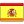## Experimental Modal Analysis

The aim of modal analysis in structural mechanics is to determine the natural frequencies, modes and parameters such as the vibration damping factor of a part  and/or structure during free vibration. The techniques of modal analysis assume that the system being studied remains invariant over time, and in turn, can be considered a linear system.

All structures have natural frequencies and a mode of vibration  associated with each. These natural frequencies depend on parameters such as the mass of the system, the stiffness or the damping of the system. In the design of machines and structures, it is necessary to identify the natural frequencies to know what the response of the designed element will be when a frequency-driving force acting on it is near to this natural frequency, or simply, what will be the response to the excitation forces that a machine or structure can suffer during its useful life.

By means of an Experimental Modal Analysis it is possible to determine natural frequencies and vibration modes of  machines  and structures. The data thus obtained can be used to solve a resonance problem or can serve to feed back a Finite Element Model, allowing for calibration of the model and the hypotheses that were used to create it. In many cases, the results and decisions that are made on the basis of the mathematical models depend on the accuracy of the feedback used in the calculation.

Modal analysis is efficient means to describe, understand and model the behavior of a structure or machine.

The following video shows a simulation performed on a fan structure: